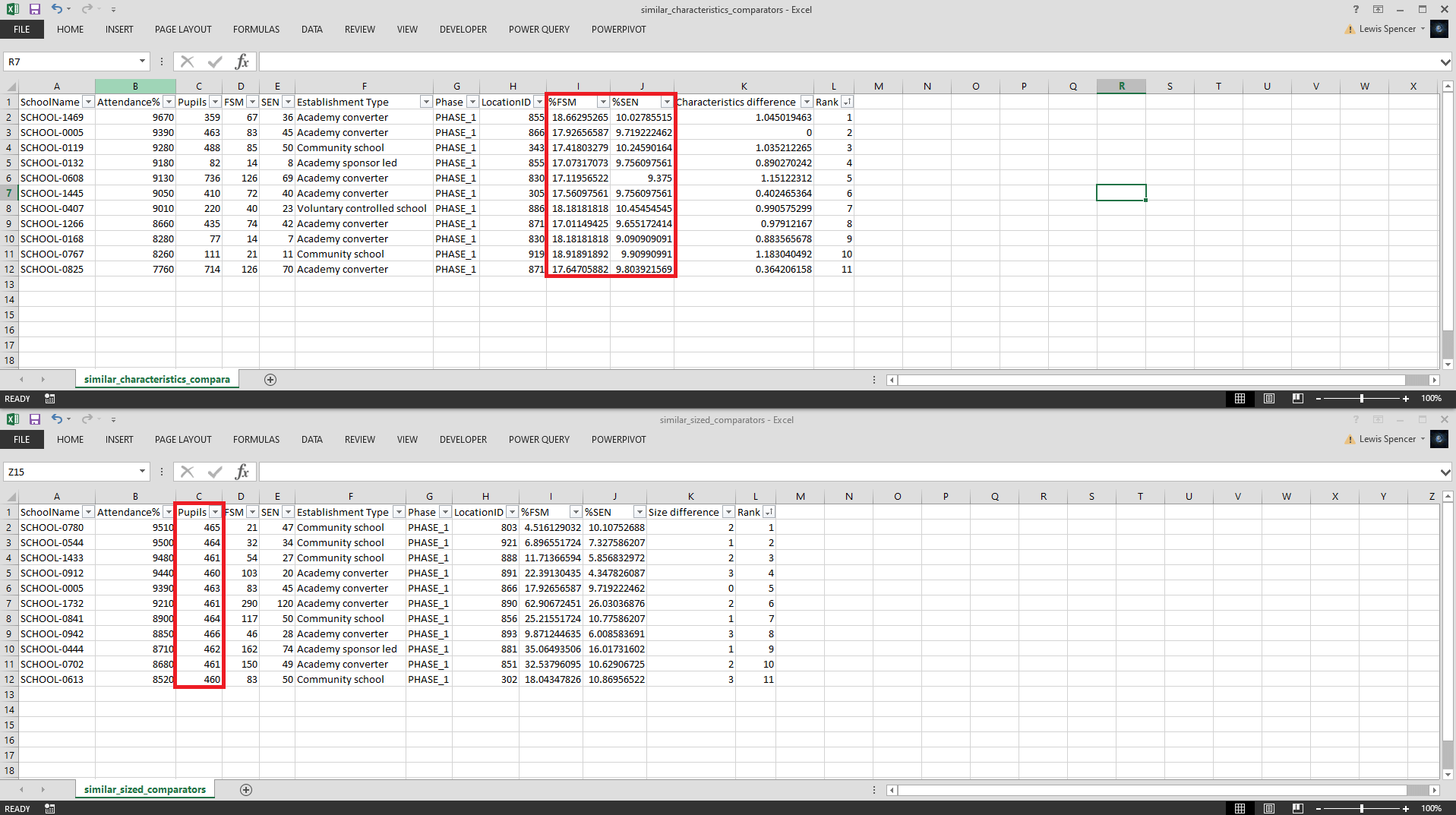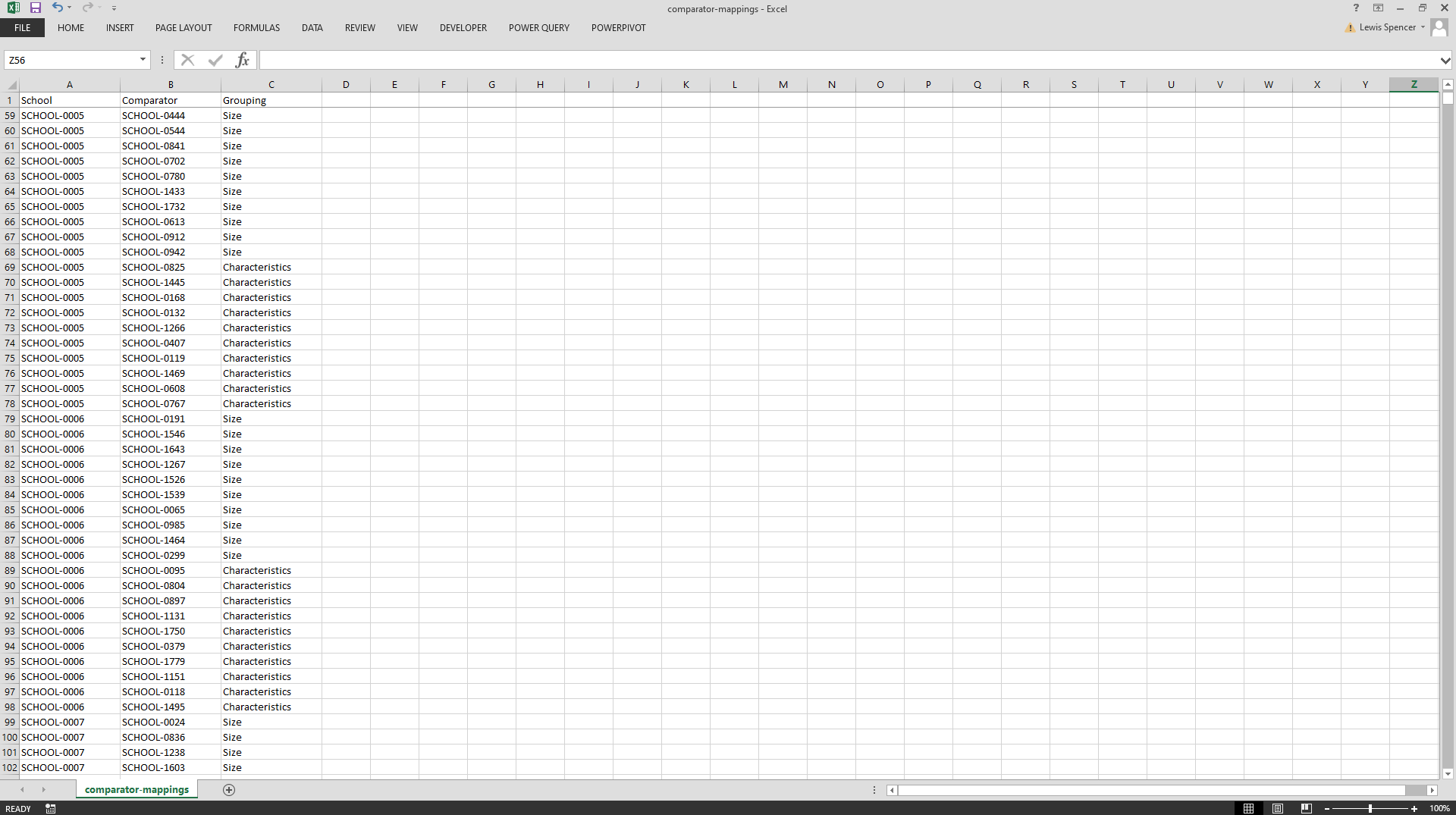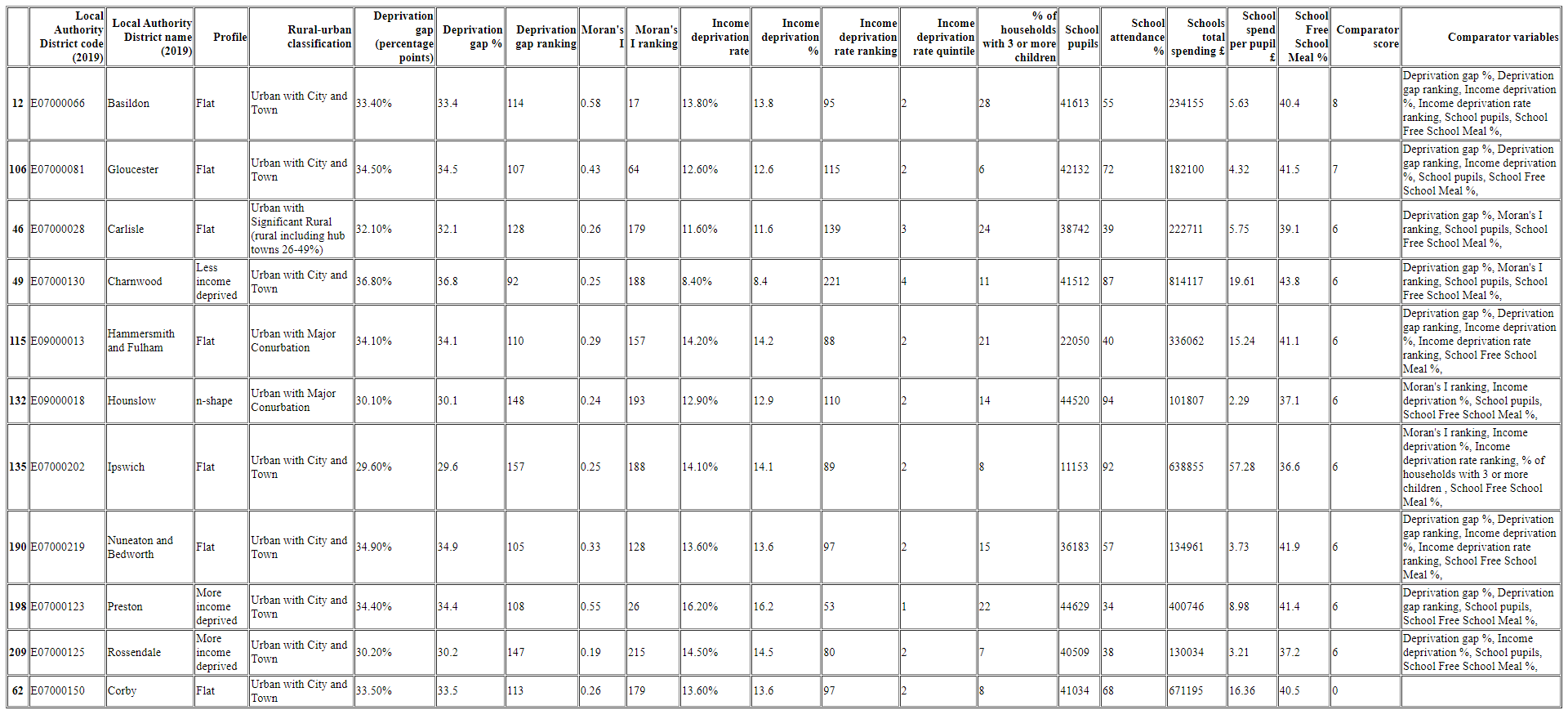Back

# Creating statistical neighbours comparator benchmarking models with Python

November 23, 2022 10 minute read

Contents

This article will explore how to get started creating a statistical neighbours model to benchmark, compare and find similar observations within a dataset. This might be comparing the sales of a store, to only other stores that are statistically similar in terms of size, budget and staffing or comparing school attendance performance for a given area to only other areas of similar size, pupil numbers and other characteristics.

The main problem of comparator models is how to define what is considered statistically 'similar'. We will explore two approaches to solving this problem.

## Filtering approach

In this dummy dataset school_data.xlsx I adapted from two good open data sources Explore education statistics and Get Information about Schools there are around 1,800 schools but we only want to compare a school's attendance levels to it's top ten most statistically similar in terms of pupil size, alongside FSM and SEN characteristics.

SchoolAttendance%PupilsFSMSENPhaseLocationID
SCHOOL-000198.26356PHASE-1855
SCHOOL-000281122925772PHASE-2873
SCHOOL-000394.82501016PHASE-1891
SCHOOL-000494.56537889PHASE-1856
SCHOOL-000593.94638345PHASE-1866
SCHOOL-000694.2918156131PHASE-2865
SCHOOL-00070812518PHASE-2888
SCHOOL-000891.41958329PHASE-1888
SCHOOL-000996.52238963PHASE-1888
SCHOOL-001092.5719253130PHASE-2209
.....................

For each school, we will apply a series of filters to find it's top ten comparators in terms of both pupil size and characteristics like FSM and SEN.

"attendance_comparators.py"
``````"""
A model to identify school comparator's based on their size and
characteristics in order to compare attendance performance.

Assumptions:

- Schools will only be compared to schools of the same phase type​.
- Results will be the top ten statistically closest schools.
- The comparators will be based on attendance %​.

Functionality:

- Ability to compare against schools of a similar size​.
​- Ability to compare against schools with similar characteristics
"""

import os
import time
import pandas as pd

def get_data() -> pd.DataFrame():
"""
Reads the Excel dataset into a Pandas DataFrame and adds new features such
as %FSM and %SEN.
"""
df["Attendance%"] = df["Attendance%"] * 100
df["%FSM"] = (df["FSM"] / df["Pupils"]) * 100
df["%SEN"] = (df["SEN"] / df["Pupils"]) * 100

return df

def generate_all_comparators(output_all_to_csv: bool = False) -> None:
"""
Generates the top 10 comparators for every school in the dataset, for each
of the 2 comparator groups (size, characteristics).

Optionally saves the result to CSV files where the folder name is the name
of the school where output_all_to_csv is set to True.
"""
df = get_data()
df_length = len(df)
comparator_mappings = []

for index, row in df.iterrows():
school_name = row["SchoolName"]

similar_sized_comparators = find_similar_sized_comparators(
school_name=school_name,
df=df
)

similar_characteristics_comparators = find_similar_characteristics_comparators(
school_name=school_name,
df=df
)

comparators=similar_sized_comparators,
mappings=comparator_mappings,
school_name=school_name,
grouping="Size"
)

comparators=similar_characteristics_comparators,
mappings=comparator_mappings,
school_name=school_name,
grouping="Characteristics"
)

if output_all_to_csv:
if not os.path.exists("output"):
os.mkdir("output")

school_name = school_name.replace("/", "")
directory = f"output/{school_name}"

if not os.path.exists(directory):
os.mkdir(directory)

similar_sized_comparators.drop(
columns=["Unnamed: 0"], inplace=True)

similar_characteristics_comparators.drop(
columns=["Unnamed: 0"], inplace=True)

similar_sized_comparators.to_csv(
directory + "/similar_sized_comparators.csv",
index=False
)
similar_characteristics_comparators.to_csv(
directory + "/similar_characteristics_comparators.csv",
index=False
)

print(f"{index + 1} of {df_length} done.")

return pd.DataFrame.from_records(comparator_mappings)

mappings,
school_name,
grouping) -> None:
"""
Builds the final output by adding all of the comparators from
the size and characteristics DataFrames to the mapping list
in JSON / dictionary format:

[
{
"School": "A",
"Comparator": "B",
"Grouping": "Size"
},
{
"School": "B",
"Comparator": "C",
"Grouping": "Characteristics"
},
]

Which results in the final output:

School   Comparator  Grouping
A        B           Size
A        D           Size
B        D           Characteristics

Avoids adding the target school_name as it's own comparator.
"""
for index, row in comparators.iterrows():
comparator_school_name = row["SchoolName"]
if comparator_school_name != school_name:
mappings.append({
"School": school_name,
"Comparator": comparator_school_name,
"Grouping": grouping
})

def find_similar_sized_comparators(school_name: str, df: pd.DataFrame) -> pd.DataFrame:
"""
Finds schools of a similar size and returns as comparators.

This comparator is calculated by the total number of pupils in each school,
per organisation type. The groupings for each organisation type will be
calculated based on the highest and lowest pupil count for schools in that
category i.e. within a given % threshold
"""
school = df[df["SchoolName"] == school_name]
school_size = school["Pupils"].values
school_type = school["Phase"].values
schools_with_same_type = df[df["Phase"] == school_type]

upper_size_threshold = school_size * 1.25
lower_size_threshold = school_size * 0.75

schools_of_similar_size = schools_with_same_type[
(schools_with_same_type["Pupils"] >= lower_size_threshold) &
(schools_with_same_type["Pupils"] <= upper_size_threshold)
].copy(deep=True)

schools_of_similar_size["Size difference"] = (abs(
schools_of_similar_size["Pupils"] -
school_size
))

schools_of_similar_size = schools_of_similar_size.nsmallest(
11, "Size difference")

schools_of_similar_size["Rank"] = (
schools_of_similar_size["Attendance%"].rank(
ascending=False
)
)

return schools_of_similar_size

def find_similar_characteristics_comparators(school_name: str, df: pd.DataFrame) -> pd.DataFrame:
"""
Finds schools with similar %FSM and %SEN characteristics and returns as comparators.
"""
school = df[df["SchoolName"] == school_name]
school_type = school["Phase"].values
school_fsm_percentage = school["%FSM"].values
school_sen_percentage = school["%SEN"].values
schools_with_same_type = df[df["Phase"] == school_type]

upper_fsm_threshold = school_fsm_percentage * 1.1
lower_fsm_threshold = school_fsm_percentage * 0.9

upper_sen_threshold = school_sen_percentage * 1.1
lower_fsm_threshold = school_sen_percentage * 0.9

schools_with_similar_characteristics = schools_with_same_type[
(schools_with_same_type["%FSM"] >= lower_fsm_threshold) &
(schools_with_same_type["%FSM"] <= upper_fsm_threshold) &
(schools_with_same_type["%SEN"] >= lower_fsm_threshold) &
(schools_with_same_type["%SEN"] <= upper_sen_threshold)
].copy(deep=True)

schools_with_similar_characteristics["Characteristics difference"] = (
abs(schools_with_similar_characteristics["%FSM"] - school_fsm_percentage) +
abs(schools_with_similar_characteristics["%SEN"] -
school_sen_percentage)
)

schools_with_similar_characteristics = schools_with_similar_characteristics.nsmallest(
11,
"Characteristics difference"
)

schools_with_similar_characteristics["Rank"] = (
schools_with_similar_characteristics["Attendance%"].rank(
ascending=False
)
)

return schools_with_similar_characteristics

if __name__ == "__main__":
start = time.time()

output = generate_all_comparators(
output_all_to_csv=True
)

output.to_csv("output/comparator-mappings.csv", index=False)

end = time.time()

print(f"Model finished in {round(end - start, 2)} seconds.")
``````

If the `output_all_to_csv` flag is set to True then for each school a folder will be created in the `output` directory for it, containing all of it's comparators for both size and pupil characteristics. An example of one of these outputs for 'SCHOOL-005' can be seen in the image below.We can see within `similar_characteristics_comparators.csv` the %FSM and %SEN are within the upper and lower thresholds and within `similar_size_comparators.csv` Pupils are within the upper and lower thresholds. This shows the model is accurately filtering and ranking only those observations that fit inside these parameters.

Within the `output` directory, there is also the full list of comparators in the `comparator-mappings.csv` file.If we also had columns for 'Easting' and 'Northing' for these schools, we could also add another filter to find the top ten geospatially closest schools.

"attendance_comparators.py"
``````from scipy.spatial import distance

def find_similar_location_comparators(school_name: str, df: pd.DataFrame) -> pd.DataFrame:
"""
Finds schools which are geospatially closest and returns as comparators.
"""
school = df[df["School"] == school_name]
school_location_id = school["LocationID"].values
school_type = school["Phase"].values
schools_with_same_type = df[df["Phase"] == school_type]
school_easting = school["Easting"].values
school_northing = school["Northing"].values

location_data_available = (
(school_easting != 0) &
(school_northing != 0)
)

if location_data_available:
geo_comparators = schools_with_same_type \
.copy(deep=True) \
.reset_index()

distances = []

for _, row in geo_comparators.iterrows():
a = (school_easting, school_northing)
b = (row["Easting"], row["Northing"])
distances.append(
distance.euclidean(a, b)
)

geo_comparators["distance"] = pd.Series(distances)
geo_comparators = geo_comparators[
geo_comparators["Phase"] == school_type
]
geo_comparators = geo_comparators.sort_values(
by="distance",
ascending=True
)

return geo_comparators

schools_in_same_area = schools_with_same_type[
(schools_with_same_type["LocationID"] == school_location_id)
].copy(deep=True)

if len(schools_in_same_area) <= 11:
return schools_in_same_area

sample =  schools_in_same_area.sample(n=10)
sample = sample.append(school)

return sample
``````

## Scoring approach

In the next example, our dummy dataset la_data.csv (adapted from a dataset taken from the ONS) is at Local Authority (area) level.

Local Authority District code (2019)Local Authority District name (2019)ProfileRural-urban classificationDeprivation gap (percentage points)Deprivation gap %Deprivation gap rankingMoran's IMoran's I rankingIncome deprivation rateIncome deprivation rate rankingIncome deprivation rate quintile% of households with 3 or more childrenSchool pupilsSchool attendance %Schools total spending £School spend per pupil £School Free School Meal %
E07000026AllerdaleFlatMainly Rural (rural including hub towns >=80%)36.60%36.60950.2915712.10%130316404616986957221.4943.60
E07000032Amber Valleyn-shapeUrban with Minor Conurbation32.90%32.901210.2915710.90%15336229814465250528.3939.90
E07000224Arunn-shapeUrban with City and Town28.70%28.701640.3113910.40%171325344496443752912.7035.70
E07000170AshfieldMore income deprivedUrban with City and Town36.00%36.00980.1524615.20%7221193665077005082.2243.00
E07000105Ashfordn-shapeUrban with Significant Rural (rural including hub towns 26-49%)29.10%29.101600.3411611.00%150326388347161322515.7936.10
E07000004Aylesbury ValeLess income deprivedLargely Rural (rural including hub towns 50-79%)19.60%19.602640.47556.70%272522384335660984815.8726.60
E07000200BaberghLess income deprivedMainly Rural (rural including hub towns >=80%)16.90%16.902800.172348.00%23242148694531465703.0123.90
E09000002Barking and DagenhamMore income deprivedUrban with Major Conurbation25.40%25.401950.2717519.40%2012136548893261358.9232.40
E09000003Barnetn-shapeUrban with Major Conurbation31.90%31.901320.3610511.10%14831548851334484739.1838.90

We want to compare a Local Authority area to only other statistically similar areas, but not just on one factor, but many (or all) numeric factors available and score them in terms of 'closeness'. This will find the top ten closest neighbours for comparisons and benchmarking.

statistical_neighbours.py
``````import pandas as pd

def find_statistical_neighbours_for(local_authority_district_code: str) -> pd.DataFrame:
filepath_or_buffer="la_data.csv",
encoding="cp1252"
)

df["Comparator score"] = 0
df["Comparator variables"] = ""

target_la = df.loc[
(df["Local Authority District code (2019)"] == local_authority_district_code)
]

comparison_variables = {
"Deprivation gap %": 1,
"Deprivation gap ranking": 1,
"Moran's I ranking": 1,
"Income deprivation %": 1,
"Income deprivation rate ranking": 1,
"% of households with 3 or more children ": 1,
"School pupils": 2,
"School Free School Meal %": 2
}

# compare the comparator variables for each LA against the target LA and score them
for index, row in df.iterrows():
is_target_la = (
row["Local Authority District code (2019)"] == local_authority_district_code
)

if is_target_la:
continue

for variable in comparison_variables:
if variables_are_statistically_similar(target_la[variable].values, row[variable]):
df.loc[index, 'Comparator score'] = (
df.loc[index, 'Comparator score'] + comparison_variables[variable]
)

df.loc[index, 'Comparator variables'] = (
df.loc[index, 'Comparator variables'] + variable + ", "
)

return(df.nlargest(10, "Comparator score").append(target_la))

def variables_are_statistically_similar(target: float, comparator: float) -> bool:
upper_bound = target * 1.10
lower_bound = target * 0.90

comparator_is_within_range = (
comparator > lower_bound and comparator < upper_bound
)

return comparator_is_within_range

def print_attendance_comparisons(df: pd.DataFrame) -> None:
target_la = df.iloc[-1]
df = df[: -1]

la_name = target_la["Local Authority District name (2019)"]
la_school_attendance_percentage = target_la["School attendance %"]

average_comparator_attendance_percentage = df["School attendance %"].mean()

print("The average school attendance percentage of your comparator LAs was ", end="")
print(f"{average_comparator_attendance_percentage}%", end="\n")
print(f"School attendance in {la_name} was {la_school_attendance_percentage}%", end="\n")

attendance_percentage_difference = (
la_school_attendance_percentage - average_comparator_attendance_percentage
)
attendance_percentage_difference = round(abs(attendance_percentage_difference), 2)

if la_school_attendance_percentage < average_comparator_attendance_percentage:
print(
f"This is {attendance_percentage_difference} "
f"percentage points lower than your comparator LAs"
)
else:
print(
f"This is {attendance_percentage_difference} "
f"percentage points higher than your comparator LAs"
)

def print_spending_comparisons(df: pd.DataFrame) -> None:
target_la = df.iloc[-1]
df = df[: -1]

la_name = target_la["Local Authority District name (2019)"]
la_school_spending = target_la["Schools total spending £"]

average_comparator_spending = df["Schools total spending £"].mean()

print("", end="\n\n")

print("The average school spending of your comparator LAs was ", end="")
print(f"£{average_comparator_spending}", end="\n")
print(f"School spending in {la_name} was £{la_school_spending}", end="\n")

spending_difference = (
la_school_spending - average_comparator_spending
)
spending_difference = round(abs(spending_difference), 2)

if la_school_spending < average_comparator_spending:
print(f"This is £{spending_difference} lower than your comparator LAs")
else:
print(f"This is £{spending_difference} higher than your comparator LAs")

if __name__ == "__main__":
comparators = find_statistical_neighbours_for("E07000150")

print_attendance_comparisons(comparators)
print_spending_comparisons(comparators)

html_file = open("index.html", "w")
html_file.write(comparators.to_html())
html_file.close()
``````
TERMINAL
Python

The scoring model works by first assigning weights in the dictionary `comparison_variables`. Then later will check each of these to see if the `variables_are_statistically_similar()` against the target Local Authority, and if so, increment the score by the weight for each.

The scoring model then first prints some summary information to the console such as comparisons between the target Local Authority's average attendance and average spending against their comparator Local Authorities. It then outputs the comparators for the target Local Authority to a HTML file 'output.html' to see which has the highest score.The output could be made to look a little nicer with some styling via CSS, but it clearly shows that across all of the comparison variables which are the 'closest' and even has a column 'Comparator variables' to show which variables were the ones driving those scores.

The target Local Authority (in this example Corby) is at the bottom of the table to refer back to. Go ahead and try plugging in different Local Authority District Codes to the `find_statistical_neighbours_for(local_authority_district_code: str)` function to see how it performs!

## What we learned

We have covered using both filtering and scoring approaches to solving statistical neighbour problems. You can now apply these models to other problems in different domains. It is a very useful ability to only compare to other observations that are statistically similar - it makes the comparison analysis more tailored and as a result the conclusions and decisions are more relevant.

Much better to compare and benchmark observations against those with similar characteristics, else you may end up making decisions that don't really apply to the school, local authority, store, or anything else the observation may be!

I did use mostly an iterative approach whilst putting these solutions together, like looping over DataFrame rows for example. If you can think of more efficient ways to solve these statistical neighbour problems for larger datasets or have any other comparator techniques you would like to share, please post a comment in the comment section below!